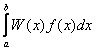Gauss-Kronrod quadrature formula is an extension of the Gaussian quadrature formula that allows for calculating integral value and estimate of its error. This is achieved by adding to M-point Gauss quadrature formula additional nodes that allow for more precise integral calculation. The difference between integral value calculated with the M-point formula and the integral value calculated using 2M+1-point formula, is used as a measure of integration error.

# Application

Due to their efficiency Gauss-Kronrod quadrature formulas are frequently used as a basic element of adaptive integrators. As a rule, the formulas used don't have high powers (usually from 15 to 60 nodes). High power formulas are not used because of the complexities that arise when calculating integrals of functions, which are not smooth enough.

Gaussian quadrature formula exists for any integral of the following type:if W(x) > 0. The nodes of quadrature formula are always located at [a,b], weight factors are strictly positive. Above said is applicable to Gauss-Kronrod quadrature formulas only in certain cases. For instance, for some W(x) functions the Gauss-Kronrod quadrature formula can have nodes beyond the boundaries of the interval [a,b] or even in complex points. Thus, when generating a formula for an arbitrary weight function W(x) please beware of the fact that for your function there might not be a suitable quadrature formula. However, in the particular case of W(x)=1 it is proven that all the formula nodes are at the integration interval and the quadrature formula can always be found.

ALGLIB Project offers you two editions of ALGLIB:

ALGLIB Free Edition:delivered for freeoffers full set of numerical functionalityextensive algorithmic optimizationsno low level optimizationsnon-commercial license

ALGLIB Commercial Edition:flexible pricingoffers full set of numerical functionalityextensive algorithmic optimizationshigh performance (SMP, SIMD)commercial license with support plan

## ALGLIB 3.16.0 for C++C++ library.
Delivered with sources.
Monolithic design.
Extreme portability.
Editions:   FREE   COMMERCIAL

## ALGLIB 3.16.0 for C#C# library with native kernels.
Delivered with sources.
VB.NET and IronPython wrappers.
Extreme portability.
Editions:   FREE   COMMERCIAL

## ALGLIB 3.16.0 for DelphiDelphi wrapper around C core.
Delivered as precompiled binary.
Compatible with FreePascal.
Editions:   FREE   COMMERCIAL

## ALGLIB 3.16.0 for CPythonCPython wrapper around C core.
Delivered as precompiled binary.
Editions:   FREE   COMMERCIAL